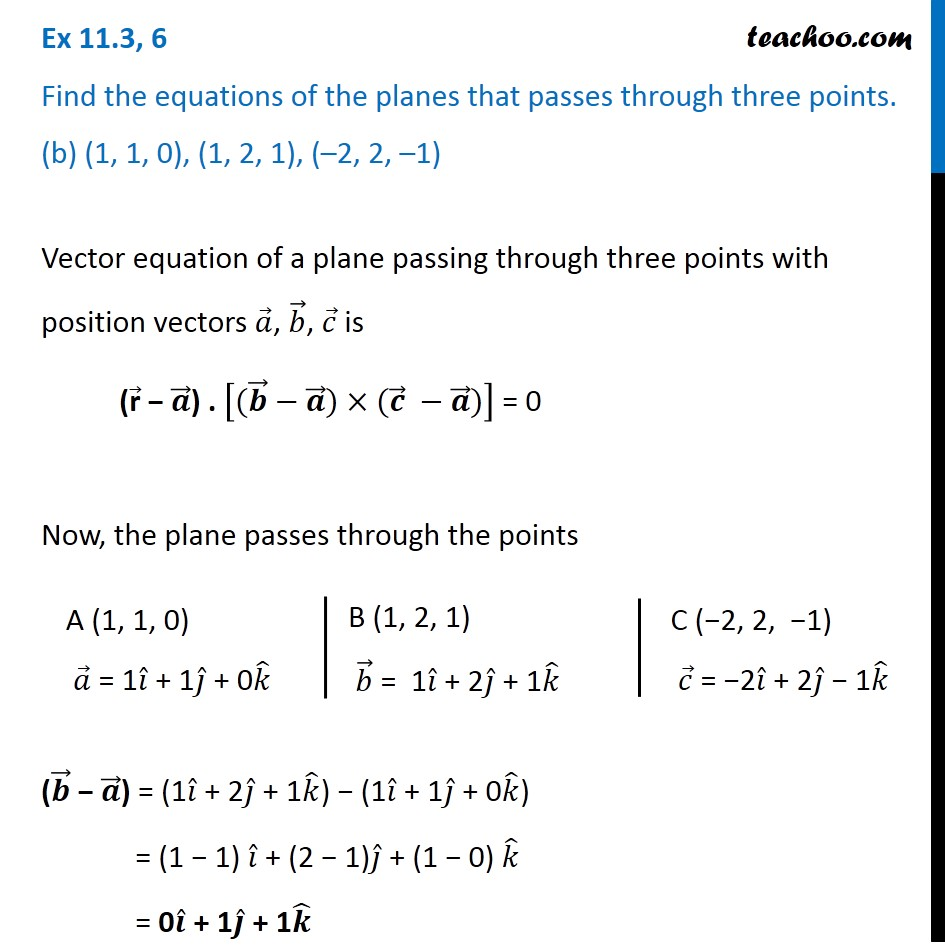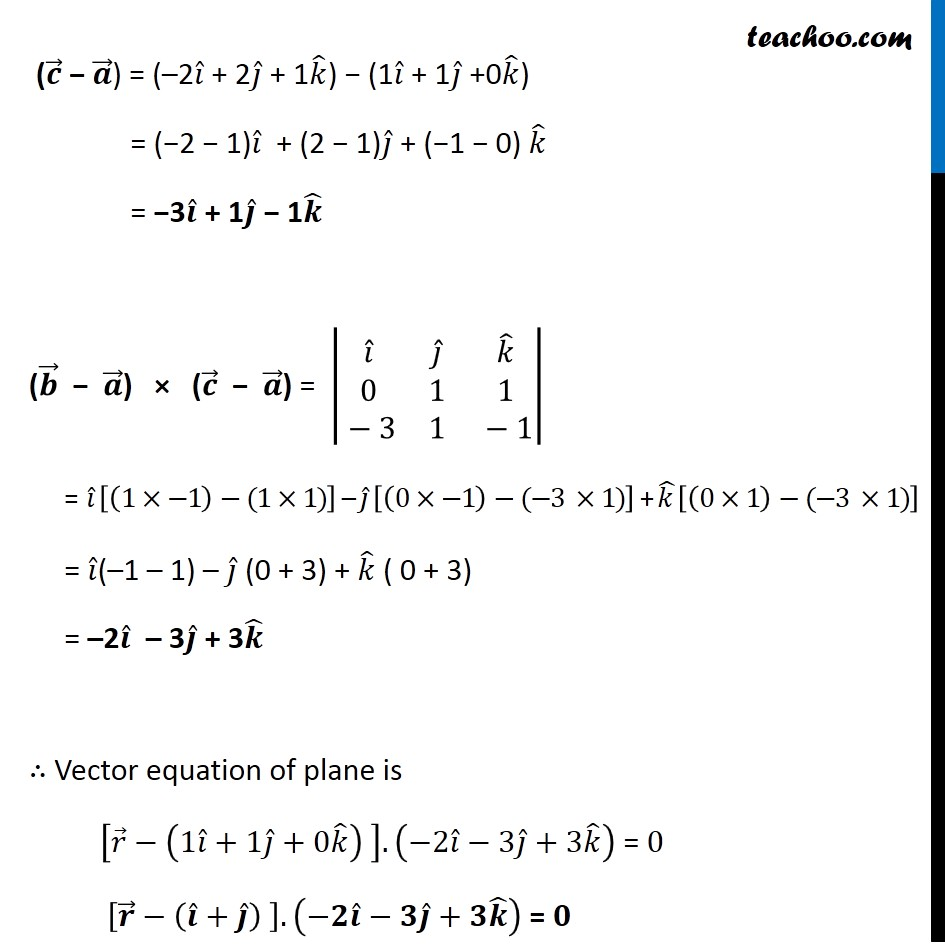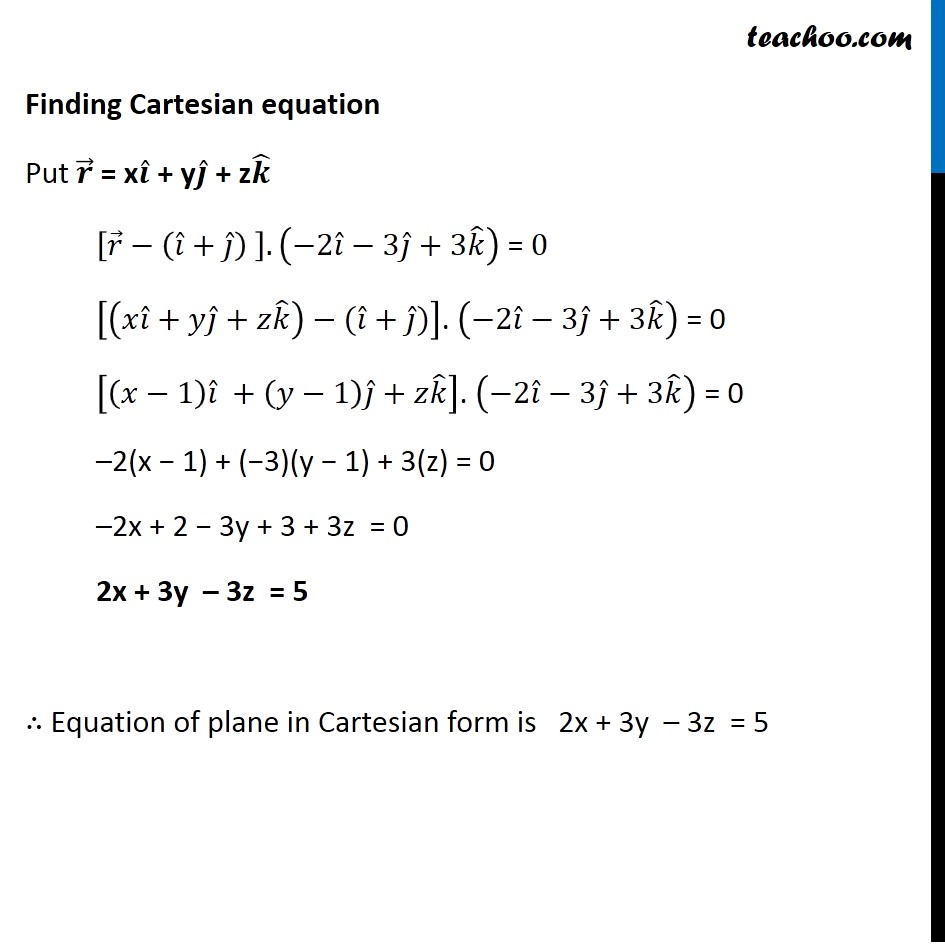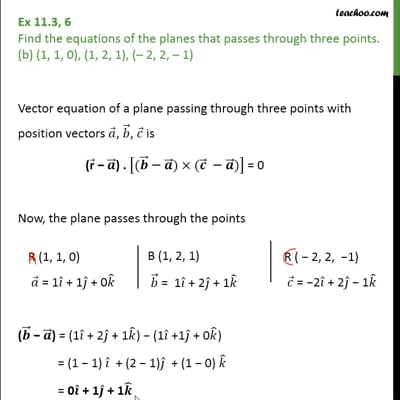Ex 11.3

Chapter 11 Class 12 Three Dimensional Geometry
Serial order wiseThis video is only available for Teachoo black users

Introducing your new favourite teacher - Teachoo Black, at only ₹83 per month

### Transcript

Ex 11.3, 6 Find the equations of the planes that passes through three points. (b) (1, 1, 0), (1, 2, 1), (–2, 2, –1) Vector equation of a plane passing through three points with position vectors 𝑎 ⃗, 𝑏 ⃗, 𝑐 ⃗ is ("r" ⃗ − 𝒂 ⃗) . [(𝒃 ⃗−𝒂 ⃗)×(𝒄 ⃗ −𝒂 ⃗)] = 0 Now, the plane passes through the points A (1, 1, 0) 𝑎 ⃗ = 1𝑖 ̂ + 1𝑗 ̂ + 0𝑘 ̂ B (1, 2, 1) 𝑏 ⃗ = 1𝑖 ̂ + 2𝑗 ̂ + 1𝑘 ̂ C (−2, 2, −1) 𝑐 ⃗ = −2𝑖 ̂ + 2𝑗 ̂ − 1𝑘 ̂ (𝒃 ⃗ − 𝒂 ⃗) = (1𝑖 ̂ + 2𝑗 ̂ + 1𝑘 ̂) − (1𝑖 ̂ + 1𝑗 ̂ + 0𝑘 ̂) = (1 − 1) 𝑖 ̂ + (2 − 1)𝑗 ̂ + (1 − 0) 𝑘 ̂ = 0𝒊 ̂ + 1𝒋 ̂ + 1𝒌 ̂ (𝒄 ⃗ − 𝒂 ⃗) = (–2𝑖 ̂ + 2𝑗 ̂ + 1𝑘 ̂) − (1𝑖 ̂ + 1𝑗 ̂ +0𝑘 ̂) = (−2 − 1)𝑖 ̂ + (2 − 1)𝑗 ̂ + (−1 − 0) 𝑘 ̂ = −3𝒊 ̂ + 1𝒋 ̂ − 1𝒌 ̂ (𝒃 ⃗ − 𝒂 ⃗) × (𝒄 ⃗ − 𝒂 ⃗) = |■8(𝑖 ̂&𝑗 ̂&𝑘 ̂@0&1&1@ − 3&1& − 1)| = 𝑖 ̂ [(1×−1)−(1×1)] – 𝑗 ̂ [(0×−1)−(−3 ×1)] + (𝑘 ) ̂[(0×1)−(−3 ×1)] = 𝑖 ̂(–1 – 1) – 𝑗 ̂ (0 + 3) + 𝑘 ̂ ( 0 + 3) = –2𝒊 ̂ – 3𝒋 ̂ + 3𝒌 ̂ ∴ Vector equation of plane is [𝑟 ⃗−(1𝑖 ̂+1𝑗 ̂+0𝑘 ̂ ) ].(−2𝑖 ̂−3𝑗 ̂+3𝑘 ̂ ) = 0 [𝒓 ⃗−(𝒊 ̂+𝒋 ̂ ) ].(−𝟐𝒊 ̂−𝟑𝒋 ̂+𝟑𝒌 ̂ ) = 𝟎 Finding Cartesian equation Put 𝒓 ⃗ = x𝒊 ̂ + y𝒋 ̂ + z𝒌 ̂ [𝑟 ⃗−(𝑖 ̂+𝑗 ̂ ) ].(−2𝑖 ̂−3𝑗 ̂+3𝑘 ̂ ) = 0 [(𝑥𝑖 ̂+𝑦𝑗 ̂+𝑧𝑘 ̂ )−(𝑖 ̂+𝑗 ̂)]. (−2𝑖 ̂−3𝑗 ̂+3𝑘 ̂ ) = 0 [(𝑥−1) 𝑖 ̂ +(𝑦−1) 𝑗 ̂+𝑧𝑘 ̂ ]. (−2𝑖 ̂−3𝑗 ̂+3𝑘 ̂ ) = 0 –2(x − 1) + (−3)(y − 1) + 3(z) = 0 –2x + 2 − 3y + 3 + 3z = 0 2x + 3y – 3z = 5 ∴ Equation of plane in Cartesian form is 2x + 3y – 3z = 5